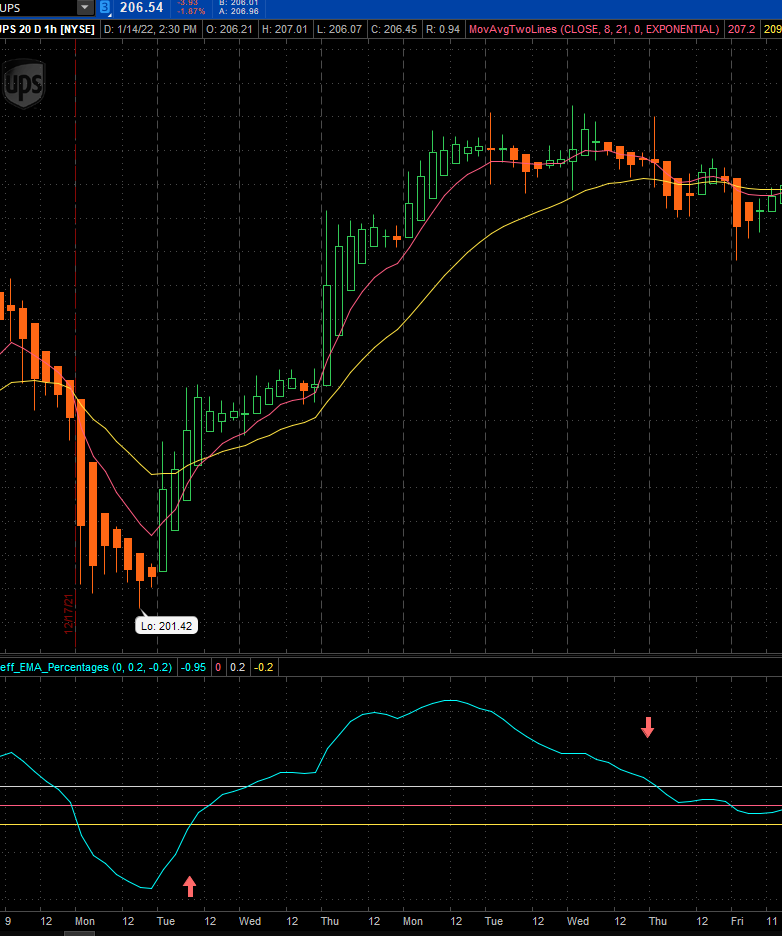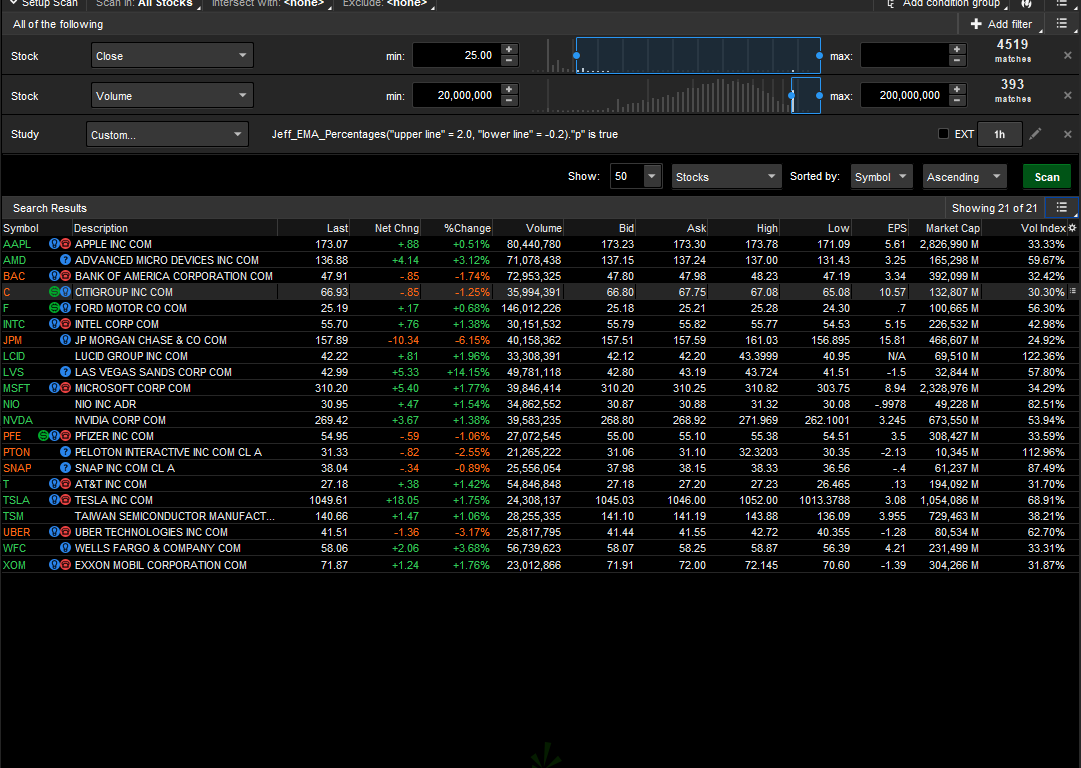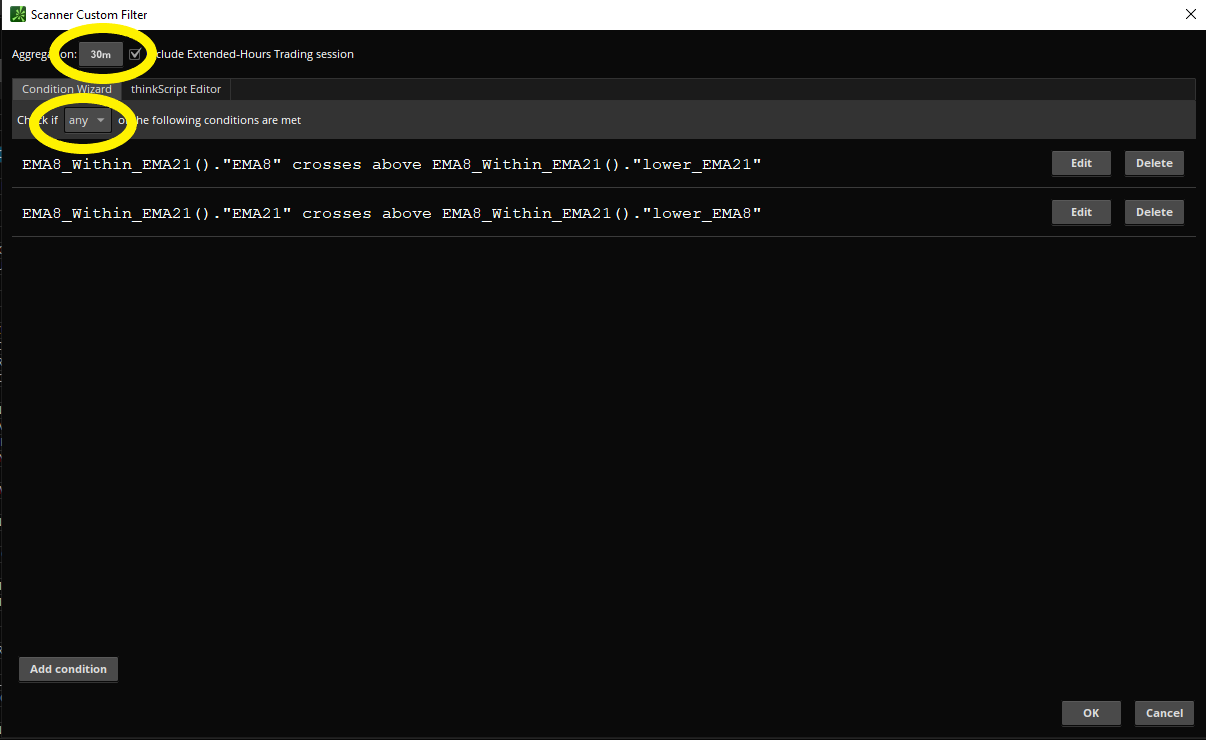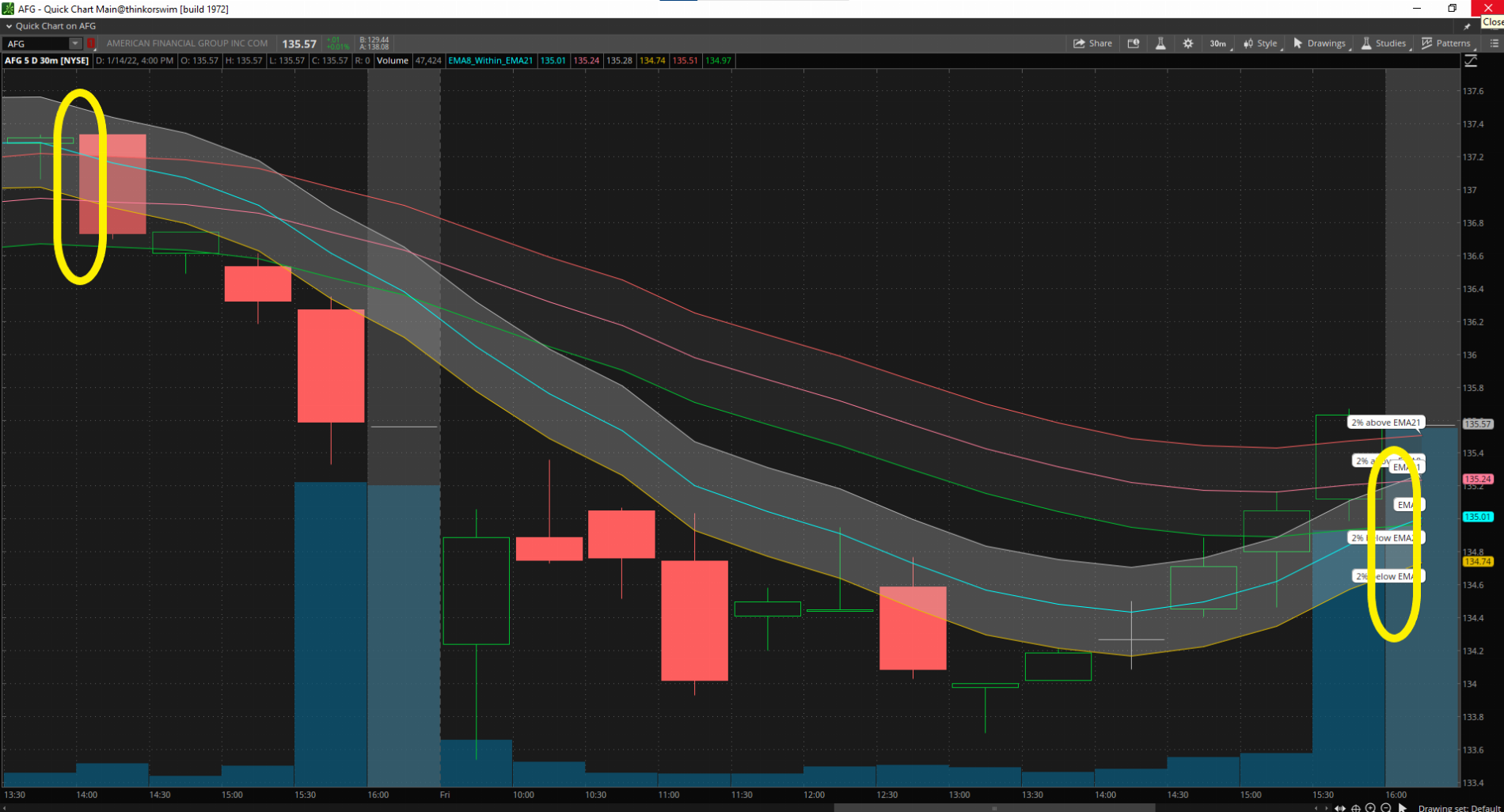# Help In Devising "Early Warning System" For Pending EMA Crossover

#### jhorton56

##### Member
VIP
I have worked all day today to try to build upon the scans posted earlier to create one that will identify stocks whose 8 EMA is rising and is within 2% of crossing its 21 EMA from below, and vice versa. I took some code from before and came up with this:

def a = ExpAverage(close, 8);
def b = ExpAverage(close, 21);
def d = a - b;
plot p = (d / b) * 100;

input center_line = 0;
plot CenterLine = center_line;

input upper_line = .2;
plot UpperLine = upper_line;

input lower_line = -.2;
plot LowerLine = lower_line;

That generates this study, with arrows pointing at the points in the price action where I would like my scan to I.D. the stocks having that characteristic (in two separate scans, one going up and v.v.).My intent is to get a jump on the time when the 8 EMA is about to cross the 21 EMA from either direction, depending on the scan I'm using. I just can't figure out after struggling for about six hours how to code it. Another way to describe it is I would like the scan to pick up on what stocks have the blue line crossing the yellow -2% line from below or the blue line crossing the white +2% line from above. The red line is where the 8 and the 21 cross. I put the study into a scan and got this, but I'm not sure if it is working how I want.•Solution
@jhorton56
Sounds like you just need to see 2% above and below the 21 EMA in your upper chart.

plot a = ExpAverage(close, 8);
plot b = ExpAverage(close, 21);
plot p1 = ((b*.2)/100)+b;
plot p2 = b-((b*.2)/100);

@jhorton56
Sounds like you just need to see 2% above and below the 21 EMA in your upper chart.

plot a = ExpAverage(close, 8);
plot b = ExpAverage(close, 21);
plot p1 = ((b*.2)/100)+b;
plot p2 = b-((b*.2)/100);

@jhorton56
Sounds like you just need to see 2% above and below the 21 EMA in your upper chart.

plot a = ExpAverage(close, 8);
plot b = ExpAverage(close, 21);
plot p1 = ((b*.2)/100)+b;
plot p2 = b-((b*.2)/100);

i think you meant to type 2 instead of .2

Actually the study up above is just for illustrative/explanatory purposes, and I do mean to use .2 for 2%, not 2 for 20%. To say in plain words, my successful scan would return a hit for a rising price EMA 8 that reached a point equal to or less than 2% away from the 21 EMA (rising blue line crossing the yellow line from below), and conversely would return a hit for a falling price 21 EMA that reached a point equal to or less than 2% away from the 8 EMA (falling blue line crossing the white line from above). See the arrows in the bottom section of my study pointing at those two cases. My objective is to become aware from alerts or through refreshing a live watchlist that one or the other of these arrow points had been reached. I understand how to scan for a price within x% of an MA, but I can't figure out how to scan for one MA being within x% of another MA. It's beyond my ability, and for all I know it can't be done.

@jhorton56
Use this code:
Code:
``````#EMA8_Within_EMA21
plot EMA8 = ExpAverage(close, 8);
plot EMA21 = ExpAverage(close, 21);

plot upper_EMA8 = ((EMA8*.2)/100)+EMA8;
plot lower_EMA8 = EMA8-((EMA8*.2)/100);

plot upper_EMA21 = ((EMA21*.2)/100)+EMA21;
plot lower_EMA21 = EMA21-((EMA21*.2)/100);

and this scan: (pay attention to aggregation period and make sure "any" is selected)will give you hits at either yellow oval:Last edited by a moderator:
Well, I'll be damned, that turned out great! Thank you very much!!!

•Well, I'll be damned, that turned out great! Thank you very much!!!
@jhorton56 are there any additional details with the moving average cross that you could share like strategy etc thanks.

I'm trying to learn how to work on a strategy of buying debit spreads based on an 8EMA-21EMA cross. Buy a put debit spread after confirming the 8 crossing down past the 21, placing one side of the spread a bit ahead of that point, going 5 to 10 wide. Opposite for a call debit spread. It occurred to me that if I can get a heads up of an imminent cross, I can watch those particular underlyings on a 60-minute chart for the cross to occur instead of scattershooting around hoping I run across a developing setup.

Cam someone share their final version of chart / scan using the TOS links? Appreciate it.

@jhorton56
Use this code:
Code:
``````#EMA8_Within_EMA21
plot EMA8 = ExpAverage(close, 8);
plot EMA21 = ExpAverage(close, 21);

plot upper_EMA8 = ((EMA8*.2)/100)+EMA8;
plot lower_EMA8 = EMA8-((EMA8*.2)/100);

plot upper_EMA21 = ((EMA21*.2)/100)+EMA21;
plot lower_EMA21 = EMA21-((EMA21*.2)/100);

and this scan: (pay attention to aggregation period and make sure "any" is selected)will give you hits at either yellow oval:Cam someone share their final version of chart / scan using the TOS links? Appreciate it.

Hey, looks like it's not the right link to the scanner, could you pass it again? Thank you
That is NOT a link to a scanner. It is a link to a study.
• copy&paste the script into the study tab
• follow the directions provided in the post that you quoted to set up the scanner.

•halcyonguy

### Not the exact question you're looking for?

87k+ Posts
315 Online## The Market Trading Game Changer

Join 2,500+ subscribers inside the useThinkScript VIP Membership Club
• Exclusive indicators
• Proven strategies & setups
• Private Discord community
• Exclusive members-only content
• 1 full year of unlimited support

What is useThinkScript?

useThinkScript is the #1 community of stock market investors using indicators and other tools to power their trading strategies. Traders of all skill levels use our forums to learn about scripting and indicators, help each other, and discover new ways to gain an edge in the markets.

How do I get started?

We get it. Our forum can be intimidating, if not overwhelming. With thousands of topics, tens of thousands of posts, our community has created an incredibly deep knowledge base for stock traders. No one can ever exhaust every resource provided on our site.

If you are new, or just looking for guidance, here are some helpful links to get you started.

What are the benefits of VIP Membership?